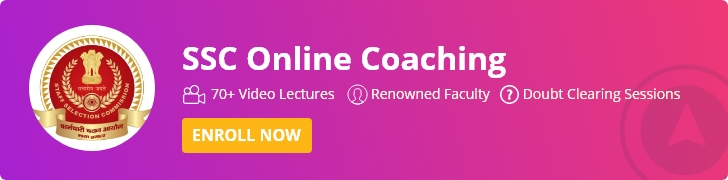# Quantitative Aptitude Preparation Tips for SSC CGL Tier I & Tier II 2020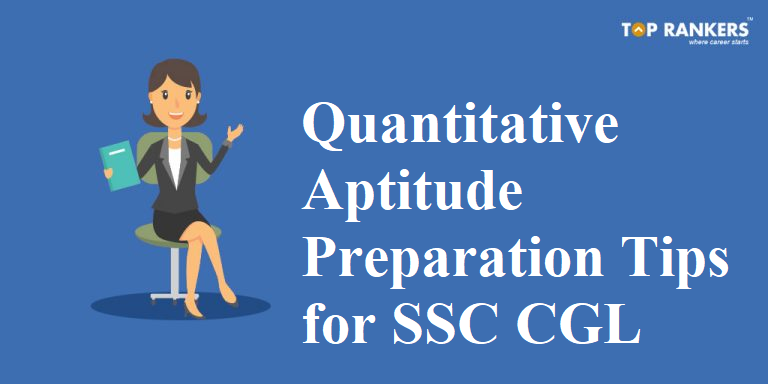Find Tips, Tricks & Strategies regarding Quantitative Aptitude Preparation Tips for SSC CGL Exam 2020 Tier I & Tier II. Get Topic wise preparation Tips with respect to the difficulty level. Go through the complete post to know Quantitative Aptitude Preparation Tips for SSC CGL Tier 1 and Tier 2.

• Begin your SSC CGL Maths Preparation for Tier 1 and 2 well in advance with experts tips and tricks. As the first stage of exam is concluded, the Tier 2 exam is scheduled in the month off June 2020.
• During preparation, candidates must keep in mind that scoring above SSC CGL Cut Off will result in getting shortlisted for the next stage of the exam.
• To ease out each one’s SSC CGL Maths preparation, we have provided preparation tips to crack SSC CGL Quantitative Aptitude. Also, taking up SSC CGL Online Coaching by experienced faculties will help in understanding the concepts in depth.

## Quantitative Aptitude Preparation Tips for SSC CGL 2020

Quantitative Aptitude is an important part of the SSC CGL examination. This particular subject is covered in Tier I & Tier II of the SSC CGL Examination process. As Quantitative Aptitude or Mathematics is a major aspect as far as preparation for SSC CGL is concerned, We have created this post to help you out with various Tips, Tricks & Strategies to have an upper hand while preparing for the Quantitative Aptitude section. For more enhanced preparation, go through SSC CGL Preparation Tips by experts to clear all stages of CGL.

• SSC CGL Tier I & II will have questions from Quantitative Aptitude.
• Various Topics such as SI/ CI, Percentage, Trigonometry, Geometry & Simplification are asked in both Tiers of SSC CGL.
• This particular subject can fetch a lot of marks if the concepts are clear properly.
• Refer NCERT Books from 9th, 10th, 11th & 12th standard.
• Look for Previous Year Question Papers & Exam Analysis for reference.
• Check out the SSC CGL Syllabus 2020 to know which topics are important from each section.

### SSC CGL Maths Preparation for Tier 1 & Tier 2

Here are the list of questions asked in the SSC CGL Tier 1 Exam 2020 Maths section. Go through these questions and be well prepared for the examination.

• a+b+c=9 ,ab=-22. find a^3+b^3+c^3-3abc
• Area of an equilateral triangle is 36root3 then find the perimeter of the same
• Given 2A=5B=7C and total amount of 1180 is divided among them. Then find the share of A?
• The area of a circle is 0.64 pie m2. In how many rotations the circle cover the distance of 1.948 km2
• Given 3a= 27b = 81c and ABC is 144 then find 2{(1/a)+(1/5b)+(1/10c)}
• A distance of 1.95 km covered by the speed of 4km/hr. If time is decreased by 25% then find the percentage increase in speed to cover the distance on time
• X+1/x =2 , find x3+1/x3
• find the value of (sin 30.sin 60)/(cos60.cos30) – tan45
• h^4+y^4 + x^2y^2 =273
• x^2-xy+y^2= 13, then find the value of xy?
• If the radius is increased by 10%, height decrease by 20%, then the volume will be?
• 5sinQ-12cosQ= 0, Then find (1+sinQ+cosQ)/(1-sinQ+cosQ)?
• 20x^2-30x+1=0, then find 25x^2+(16x^2)^-1
• If CP is increased by 25%, then x% discount provided by the shopkeeper. Find loss?
• 30×2 -15x +1 =0. Find 25×2 + x2/36
• Find the value sec6x -tan6x +3sec2x.tan2x
• 2 numbers with divisibility from 11 and 72 were given. 5x-6y value?
• Question asked in the triangle -Exterior angle bisector
• DI was tabular on 4 companies production of motorcycle
• (Ax²+y²+Bz)² = 4x² + y² + 8z² + 2 (2xy + 2 root 2 yz + 4 root 2 xz). Find A² + B² – AB
• The number between 200 and 800. Which are neither divisible by 5 nor 7
• If the breadth of a rectangle is increased by 40% and length is increased by some %, Then find the percentage change in the area of the rectangle?
• If x^2a = y^2b=z^2c , then x²/y² =?
• There are 640 students, the number of boys = 400, the number of girls =240. If 30 Girls added then how many boys should be added to make the ratio of boy to girl 14:9?
• If A = 4 sin a , B = 5 cos b , Then Find A^2 + B^2
• x²+3x+1=7 find x^6+1/x^6
• The average of 4 numbers is 3 times the 5th number. Average of 5 numbers were given. Find 5th number
• 2sin@+ 15cos2@=7 , find tan@+cos@+sec@=?
• DI based on 4 companies and their data from 2014-2019
• Average of 5 consecutive even number is m. If the next 5 consecutive even numbers is added. Then find its average?
• Find the value of tan60+cosec60=?
• In a triangle ABC, AB=AC, Angle A=40. Then find the value of B?
• In a race A runs at 100m/min, B runs at 120m/min. B looses to A by 10 min, then find the total distance?
• A can complete a work in 20 days, B can do it in 25 days. They both work for 5 days, then B left. In how will days A will complete the remaining work?
• Each question has separate DI.

### Quantitative Aptitude Preparation Tips for SSC CGL Tier I & Tier II

SSC CGL Tier I examination Consists of  4 subjects for a total of 200 questions for 200 marks. Quantitative Aptitude consists of 50 questions for 50 Marks. There are various topics that are covered during the Quantitative Aptitude examination for SSC CGL Tier I.

In the SSC CGL Tier II examination, Quantitative Aptitude carries 200 marks from 100 questions. It consists of similar topics as in Tier I examination. Now, Let’s breakdown all the Quantitative Aptitude topics on the basis of their difficulty level. To analyze your SSC CGL Maths preparation levels, it is suggested to attempt the SSC CGL Mock Test Series regularly.

#### ⇒Difficulty Level:- Easy

• Do not get stuck in one type of problem, look for new types and find quicker ways to solve the problem.
• Make sure you know the SSC CGL Exam Pattern 2020 correctly.
• Write down all the basic formulae and other important details related to these topics for convenience.
• Well, practice is the key. Revise as much as you can to avoid any mistake.
 Topics No of Questions (Tier-I) No of Questions (Tier-II) Remark Simplification 1-2 Qs 5-6 Qs Learn fast calculation simplification techniques based on BODMAS, Fractions, Surds, and Indices, etc. Interest 1-2 Qs 5-6 Qs Go through the theory of Interest and solve SI Problems on the different rates of interest. Use tricks to reduce solving time. Percentage 1 Qs 2-3 Qs Practice calculating the percentage of a given number mentally without using pen & paper. This will improve your calculation speed Average 1 Qs 4-5 Qs Learn the basics and start solving  questions on Average Ratio & Proportion 1-2 Qs 2-3 Qs Practice questions based on Ratios & Proportions and understand the pattern in which the questions are asked. Problems on Ages 1 Qs 1-2 Qs Start with solving the basic questions and then move to more complex problems.

#### ⇒Difficulty Level:- Moderate

• This part of the quantitative aptitude is full of lengthy calculations. Try learning tricks in order to save time.
• Formulae must be written down and one should always carry it unless he/she remembers all of it.
• Data Interpretation can fetch you good marks. Try different types of Bar Graph, Pie Chart questions to make the most out of this section.
• Have a look at the below table for gearing up your SSC CGL Maths Preparation. Go through the number of questions asked from each topic for both Tier 1 and Tier 2.
 Topics No. of Questions (Tier I) No. of Questions (Tier II) Remark Speed Time and Distance 1-2 Qs 5-6 Qs Solve and practice problems based on Average/ Relative speeds, Boats & Trains Profit and Loss 2 Qs 4-6 Qs Follow the basic concepts and solve the problem based on Dishonest/ Successive dealings, Partnership etc. Number Series 1 Qs 2-3 Qs Solve different types of problems from addition, subtraction, multiplication, division, square form etc. to understand the pattern well. Number System 1 Qs 5-6 Qs Practice problems based on LCM & HCF, Irrational or Rational Numbers Mensuration 2-3 Qs 7-8 Qs Remember the basic formulas of mensuration & solve problems on Square, Rectangle, Circle, Cone, cylinder by applying tricks to reduce time. Data Interpretation 2-4 Qs 5 Qs Solve and practice problems based on Bar Graphs, Pie Charts, Tables etc.

#### ⇒Difficulty Level:- Difficult

• Geometry has the maximum weight-age of all the topics mentioned here.
• Generally, Geometry is a bit tricky but a regular revision can make you aware of the important details.
• Practice all the trigonometric identities and solve as many questions as possible to understand the pattern of the different type of questions.
• Here is the tabular column for SSC CGL Quantitative Aptitude Preparation. Know the number of questions asked from each topic in the Tier and Tier 2 Maths section.
 Topics No. of Questions (Tier I) No. of Questions (Tier II) Remark Time and Work 1-2 Qs 4-5 Qs Learn Tricks for Time & work, pipes & cistern, work & efficiency and practice regularly on these topics. Mixture Problems 0-1 Qs 3-4 Qs Practice problems based on two or more entities/ mixtures. Algebra 2-3 Qs 5-7 Qs Solve Algebra problems by making Linear equations in one or two variables. Try to remember all the identities for fastening your speed Geometry 3-4 Qs 8-10 Qs Practice with problems related to Triangles congruency, similarity theorems, Circle chords & tangent theorems, Co-ordinate geometry, etc. Remember all the theorem to speed up the process. Trigonometry 2-3 Qs 4-6 Qs Practice and remember all the Trigonometric identities & solve the questions.

### Best Books – Quantitative Aptitude Preparation Tips for SSC CGL Tier I & Tier II

Finding the best book for any exam preparation can be a huge task. Well, we understand your needs and so have we brought for you a list of best books for SSC CGL Quantitative Aptitude Preparation. For proper preparation, studying from the SSC CGL Best Books are very important

• Quantitative Aptitude for Competitive Examinations by R.S. Aggarwal
• Quantitative Aptitude for CAT by Arun Sharma
• Arihant Publications – Fast Track Objective Arithmetic
• Quicker Maths by M Tyra (For beginners)
• D. Sharma – Mathematics Class 11th and 12th
• Fast Track by Rajesh Verma.

### Question Paper Type – Quantitative Aptitude Preparation Tips for SSC CGL

Knowing the Question Paper Type makes things easier for the students as it gives an idea of its difficulty level. We have provided 10 questions with their answers below;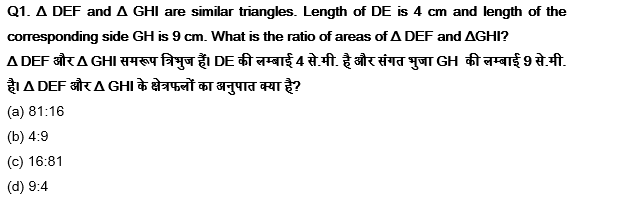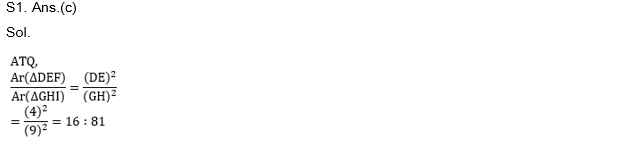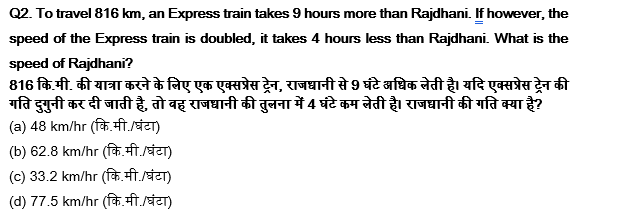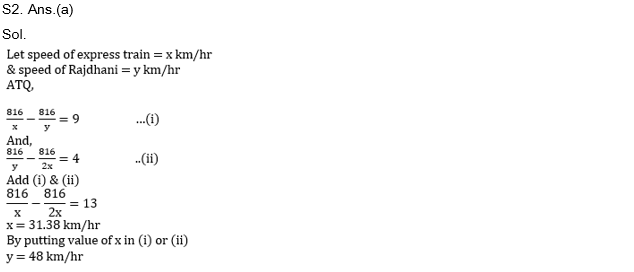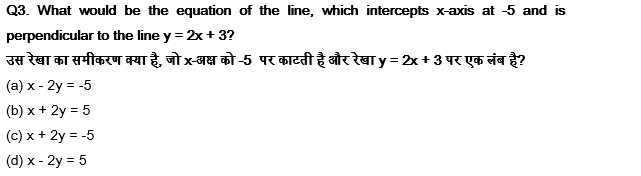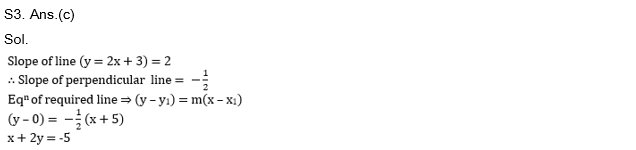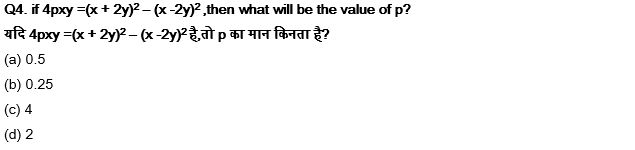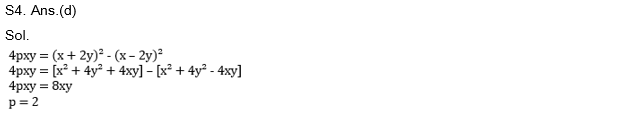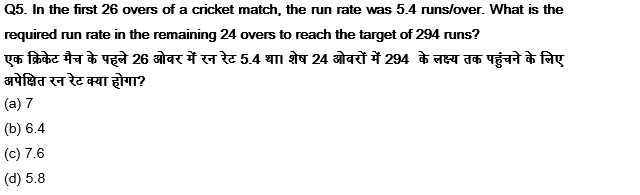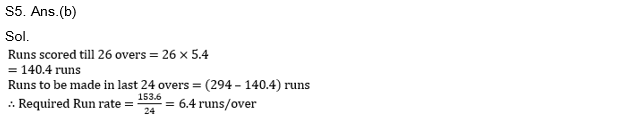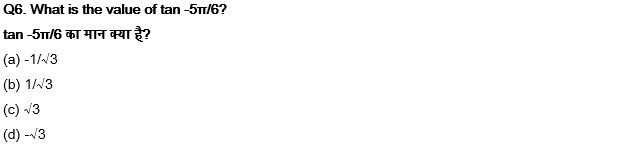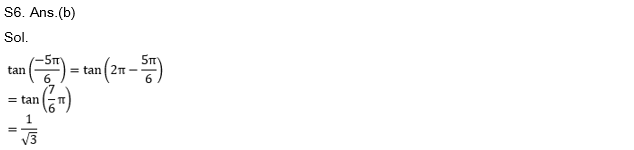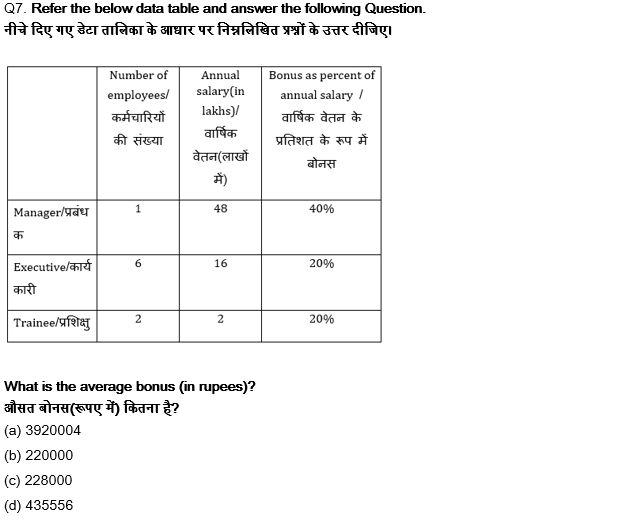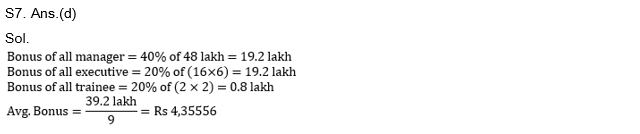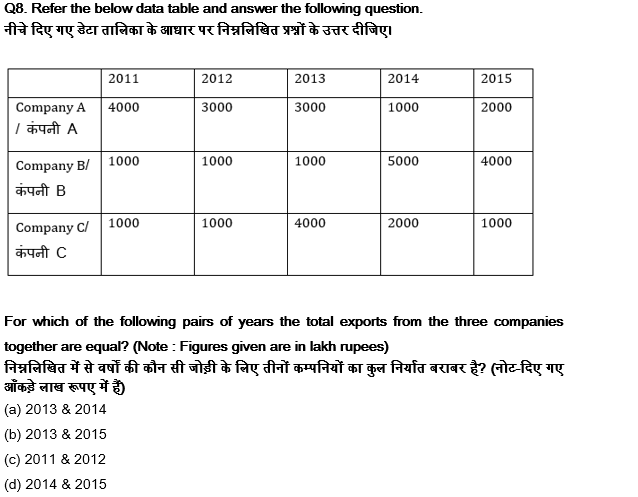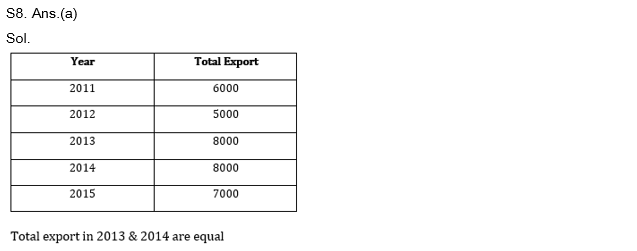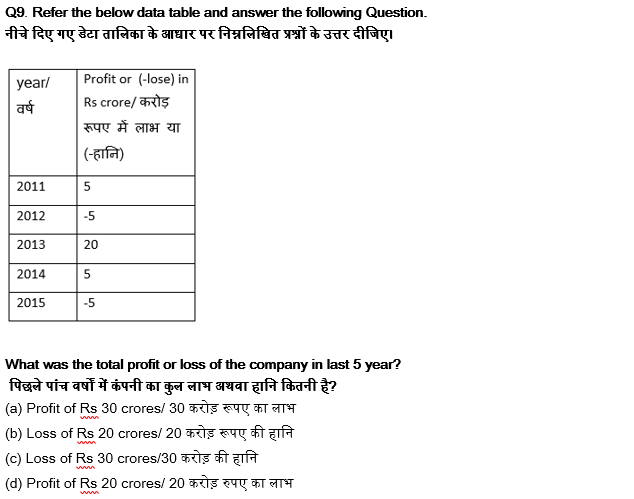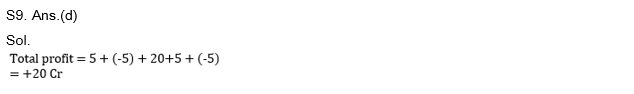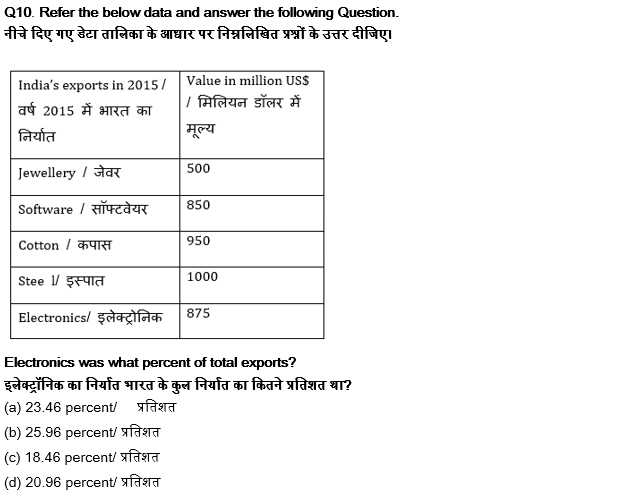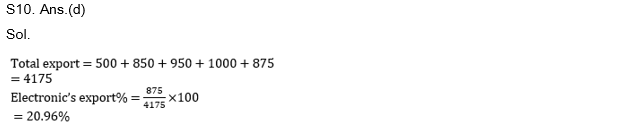Along with these questions, take up a quiz daily to enhance your preparation. Click on the below link to attempt a subject-wise quiz for SSC CGL Exam 2020.